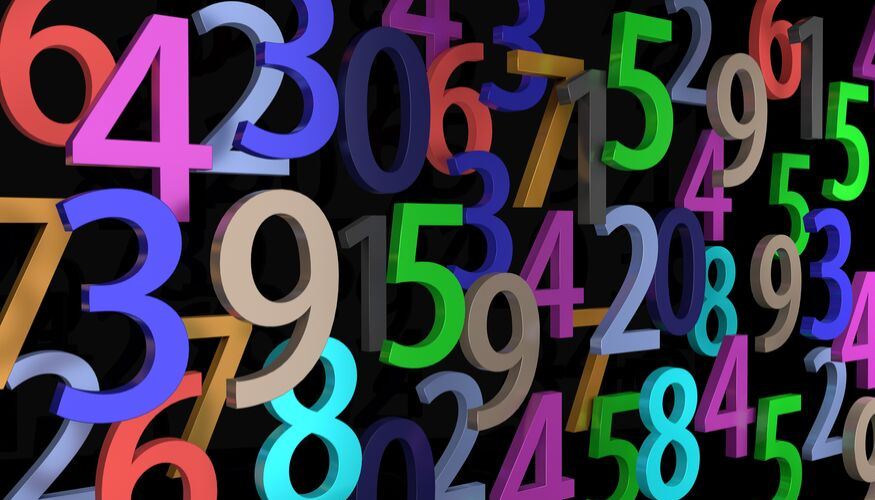# Prime Numbers: New Method To Know More About Prime Numbers?Lets Find Out How!351A new method to show the interrelation between a small and a big natural number is here! It shows the beauty of prime numbers in a fun and interactive way! A prime number is a natural number that is greater than one but cannot be formed by multiplying two smaller numbers, for example, 3,5,7, etc.

The model contains natural numbers arranged in an increasing form in which the first row contains one natural number, the second contains two, third contains three and so on. These numbers are in sequence.

In this model, three horizontal lines are drawn between the numbers. If we take a number above the line after one number up then we have to take a number below that line after one number below and then if we add the digits present in these numbers, then their summation will show similarity. This means if we take a number above the line and a different number below the same line but at an equal distance on both sides of the line then the sum of their digits will be equal.

This principle of natural number is quite a beauty and an interesting topic for mathematics lovers.

There are some advantages to this model like:

• This model can be used to find a relation between a smaller and a bigger number.
• This method will increase the understanding of prime numbers in students.
• This method is easy and great.

### Career In Relevant Field:

One can aim for career in mathematics if he/she has an interest in math. One can opt for the job of a professor in school or college. And also there are various job options for students who have a bachelor?s or master?s degree in mathematics like:

• Data Scientist
• Process Specialist - DB Operations
• Data Analyst